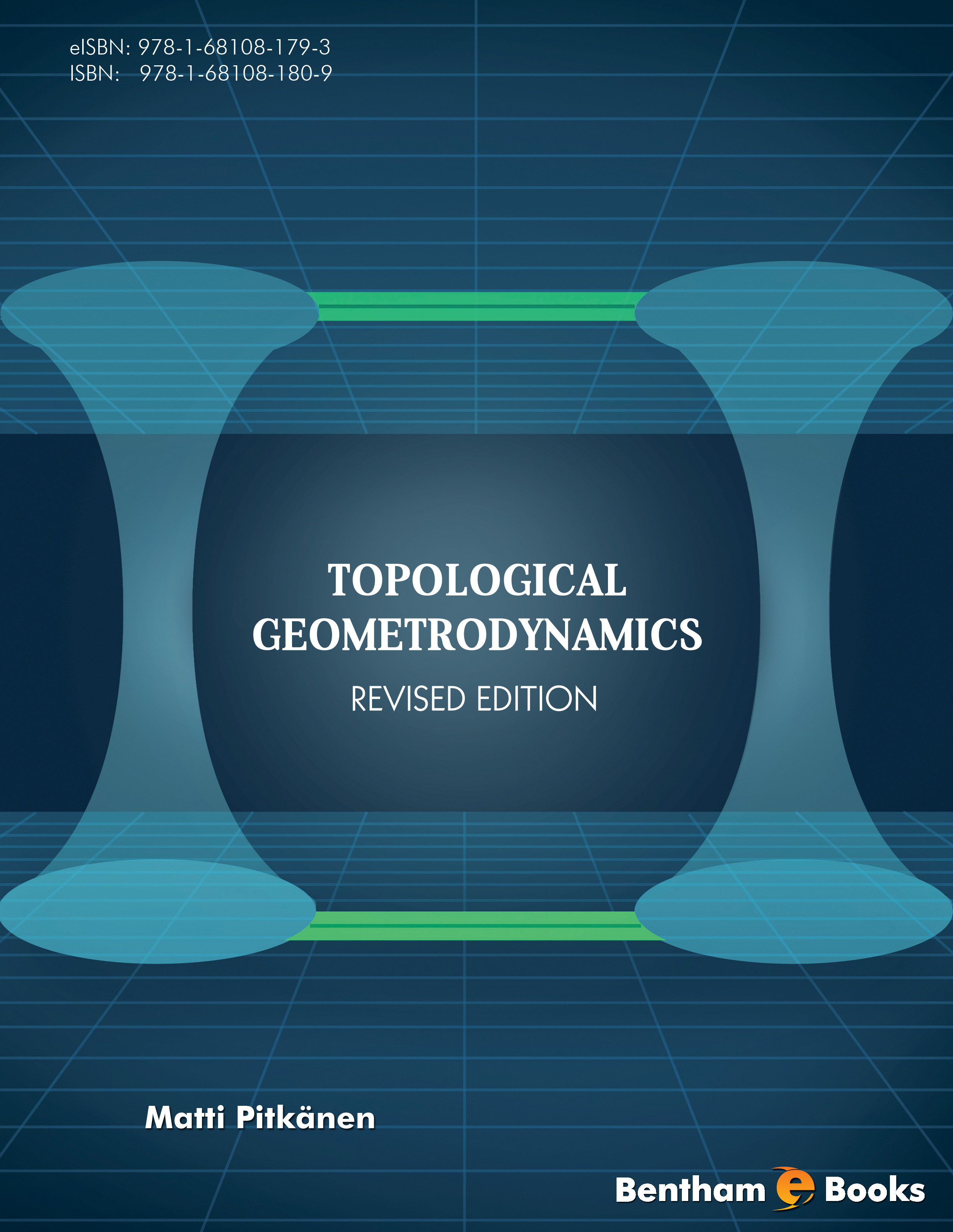### TGD From Particle Physics Perspective

#### Abstract

Topological Geometrodynamics is able to make rather precise and often testable predictions. In this and two other articles I want to describe the recent over all view about the aspects of quantum TGD relevant for particle physics. &lt;/p&gt;&lt;p&gt; In the first chapter I concentrate the heuristic picture about TGD with emphasis on particle physics. &lt;/p&gt;&lt;p&gt; 1. First I represent briefly the basic ontology: the motivations for TGD and the notion of many-sheeted space-time, the concept of zero energy ontology, the identification of dark matter in terms of hierarchy of Planck constant which now seems to follow as a prediction of quantum TGD, the motivations for p-adic physics and its basic implications, and the identi cation of space-time surfaces as generalized Feynman diagrams and the basic implications of this identification. &lt;/p&gt;&lt;p&gt; 2. Symmetries of quantum TGD are discussed. Besides the basic symmetries of the imbedding space geometry allowing to geometrize standard model quantum numbers and classical fields there are many other symmetries. General Coordinate Invariance is especially powerful in TGD framework allowing to realize quantum classical correspondence and implies effective 2-dimensionality realizing strong form of holography. Super-conformal symmetries of super string models generalize to conformal symmetries of 3-D light-like 3-surfaces. &lt;/p&gt;&lt;p&gt; What GRT limit of TGD and Equivalence Principle mean in TGD framework have are problems which found a solution only quite recently (2014). GRT space-time is obtained by lumping together the sheets of many-sheeted space-time to single piece of M&lt;sup&gt;4&lt;/sup&gt; provided by an effctive metric defined by the sum of Minkowski metric and the deviations of the induced metrics of space-time sheets from Minkowski metric. Same description applies to gauge potentials of gauge theory limit. Equivalence Principle as expressed by Einstein&#039;s equations reflects Poincare invariance of TGD. Super-conformal symmetries imply generalization of the space-time supersymmetry in TGD framework consistent with the supersymmetries of minimal supersymmetric variant of the standard model. Twistorial approach to gauge theories has gradually become part of quantum TGD and the natural generalization of the Yangian symmetry identi ed originally as symmetry of N = 4 SYMs is postulated as basic symmetry of quantum TGD......

Total Pages: 233-325 (93)